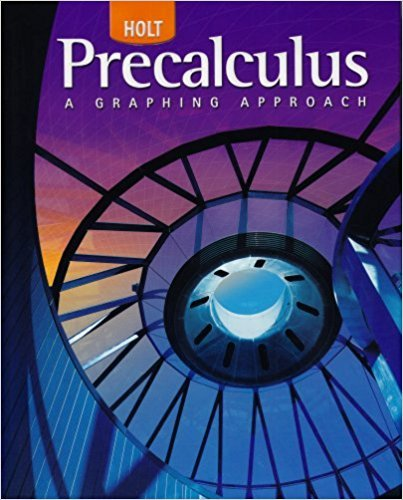×
×

# Solutions for Chapter 4.5.A: Excursion: The Mandelbrot Set## Full solutions for Precalculus | 1st Edition

ISBN: 9780030416477Solutions for Chapter 4.5.A: Excursion: The Mandelbrot Set

Solutions for Chapter 4.5.A
4 5 0 374 Reviews
20
0
##### ISBN: 9780030416477

Since 20 problems in chapter 4.5.A: Excursion: The Mandelbrot Set have been answered, more than 26031 students have viewed full step-by-step solutions from this chapter. Precalculus was written by and is associated to the ISBN: 9780030416477. Chapter 4.5.A: Excursion: The Mandelbrot Set includes 20 full step-by-step solutions. This textbook survival guide was created for the textbook: Precalculus, edition: 1. This expansive textbook survival guide covers the following chapters and their solutions.

Key Calculus Terms and definitions covered in this textbook
• Bounded interval

An interval that has finite length (does not extend to ? or -?)

• Coefficient matrix

A matrix whose elements are the coefficients in a system of linear equations

• Complements or complementary angles

Two angles of positive measure whose sum is 90°

• Component form of a vector

If a vector’s representative in standard position has a terminal point (a,b) (or (a, b, c)) , then (a,b) (or (a, b, c)) is the component form of the vector, and a and b are the horizontal and vertical components of the vector (or a, b, and c are the x-, y-, and z-components of the vector, respectively)

• Conversion factor

A ratio equal to 1, used for unit conversion

• Correlation coefficient

A measure of the strength of the linear relationship between two variables, pp. 146, 162.

• Double-angle identity

An identity involving a trigonometric function of 2u

• Graph of a function ƒ

The set of all points in the coordinate plane corresponding to the pairs (x, ƒ(x)) for x in the domain of ƒ.

• Head minus tail (HMT) rule

An arrow with initial point (x1, y1 ) and terminal point (x2, y2) represents the vector <8x 2 - x 1, y2 - y19>

• Inverse cotangent function

The function y = cot-1 x

• Median (of a data set)

The middle number (or the mean of the two middle numbers) if the data are listed in order.

• Multiplicity

The multiplicity of a zero c of a polynomial ƒ(x) of degree n > 0 is the number of times the factor (x - c) (x - z 2) Á (x - z n)

• Outliers

Data items more than 1.5 times the IQR below the first quartile or above the third quartile.

• Positive linear correlation

See Linear correlation.

• Power-reducing identity

A trigonometric identity that reduces the power to which the trigonometric functions are raised.

• Pseudo-random numbers

Computer-generated numbers that can be used to approximate true randomness in scientific studies. Since they depend on iterative computer algorithms, they are not truly random

• Speed

The magnitude of the velocity vector, given by distance/time.

• Sum of a finite arithmetic series

Sn = na a1 + a2 2 b = n 2 32a1 + 1n - 12d4,

• Vertex of a cone

See Right circular cone.

• xz-plane

The points x, 0, z in Cartesian space.

×